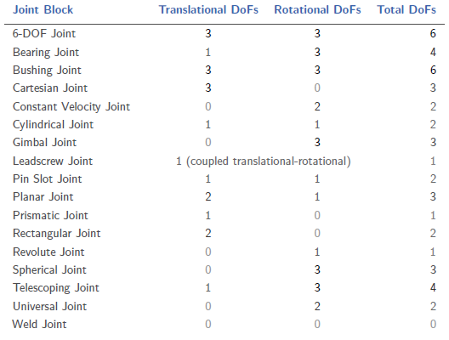## Modeling Joint Connections

Joints impose between bodies the primary kinematic constraints that determine how they can move relative to each other. A joint can be a physical connection, such as that between the case and shaft of a linear hydraulic actuator, or a virtual connection, such as that between the Earth and the moon. In Simscape™ Multibody™, you model both connection types using Joint blocks.

Examples of physical and virtual connections between bodiesGear and Constraint blocks too impose kinematic constraints between bodies. How are joint blocks different? While Gear and Constraint blocks are parameterized in terms of the DoFs they remove between bodies, Joint blocks are parameterized in terms of the DoFs they provide, through modules called joint primitives.

### Joint Degrees of Freedom

Each Joint block connects exactly two bodies. Such a connection determines the maximum degrees of freedom, or DoFs, that the adjoining bodies can share. These DoFs range from zero in the Weld Joint block to six—three translational and three rotational—in 6-DOF Joint and Bushing Joint blocks. Translation refers to a change in position and rotation to a change in orientation.

Joint DoFs are a measure of joint mobility. Precluding other constraints in a model, a joint with more DoFs allows greater freedom of motion between the adjoining bodies. Joint DoFs also have a mathematical interpretation. They are the minimum number of state variables needed to fully determine the configuration of a joint at each time step during simulation.

Consider a rectangular joint. This joint allows translation in a plane and it therefore has two translational DoFs—one for each spatial dimension. At each time step, the joint configuration is fully determined by two state variables, the position coordinates in the plane of motion [x(t), y(t)]. This means, for example, that you can fully prescribe motion at this joint using two position input signals.The table summarizes the DoFs that the various Joint blocks provide.The actual DoFs at a joint are often fewer in number than the joint alone would allow. This happens when kinematic constraints elsewhere in the model limit the relative motion of the adjoining bodies. Such constraints can arise from gears in mesh, forbidden DoFs due to other joints in closed kinematic loops, and fixed distances and angles between bodies, among other factors.

### Joint Primitives

Joint blocks are assortments of joint primitives, basic yet complete joints of various kinds you cannot decompose any further—at least without losing behavior such as the rotational-translational coupling of the lead screw joint. Joint primitives range in number from zero in the Weld Joint block to six in the Bushing Joint block. There are five joint primitives:

• Prismatic — Allows translation along a single standard axis (x, y, or z). Joint blocks can contain up to three prismatic joint primitives, one for each translational DoF. Prismatic primitives are labeled P*, where the asterisk denotes the axis of motion, e.g., Px, Py, or Pz.

• Revolute — Allows rotation about a single standard axis (x, y, or z). Joint blocks can contain up to three revolute joint primitives, one for each rotational DoF. Revolute primitives are labeled R*, where the asterisk denotes the axis of motion, e.g., Rx, Ry, or Rz.

• Spherical — Allows rotation about any 3-D axis, [x, y, z]. Joint blocks contain no more than one spherical primitive, and never in combination with revolute primitives. Spherical primitives are labeled S.

• Lead Screw Primitive — Allows coupled rotation and translation on a standard axis (e.g., z). This primitive converts between rotation at one end and translation at the other. Joint blocks contain no more than one lead screw primitive. Lead screw primitives are labeled LS*, where the asterisk denotes the axis of motion.

• Constant Velocity Joint — Allows rotation at constant velocity between intersecting though arbitrarily aligned shafts. Joint blocks contain no more than one constant velocity primitive. Constant velocity primitives are labeled CV.

The table summarizes the joint primitives and DoFs that the various Joint blocks provide.Why use Joint blocks with spherical primitives? Those with three revolute primitives are susceptible to gimbal lock—the natural but often undesired loss of one rotational DoF when any two rotation axes become aligned. Gimbal lock leads to simulation errors due to numerical singularities. Spherical primitives eliminate the risk of gimbal-lock errors by representing 3-D rotations using 4-D quantities known as quaternions.

### Joint Inertia

Simscape Multibody joints are idealized. They differ from real joints in that they have no inertia—a suitable approximation in most models, where the impact of joint inertia on system dynamics is often negligible. This is the case, for example, in the constant-velocity joints of automobile driveline systems, where shaft inertia can dwarf joint inertia.

If joint inertia is important in your model, you can account for it using Solid or Inertia blocks. Connect the block reference frame ports to the appropriate joint frames and specify the joint inertial properties in the block dialog boxes. You can specify joint mass or density, products of inertia, moments of inertia, and center of mass. For more information on how to specify inertia, see Representing Solid Inertia.